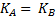# If all of a sudden the radius of the earth decreases, then a) The angular momentum of the earth will become greater than that of the sun b) The angular speed of the earth will increase c) The periodic time of the earth will increase d) The energy and angular momentum will remain constant

## Question ID - 53729 :- If all of a sudden the radius of the earth decreases, then a) The angular momentum of the earth will become greater than that of the sun b) The angular speed of the earth will increase c) The periodic time of the earth will increase d) The energy and angular momentum will remain constant

3537

 (b) If radius of earth decreases then its M.I. decreases As[constant]angular velocity of the earth will increase
 The moments of inertia of two freely rotating bodiesandareandrespectively.and their angular momenta are equal. Ifandare their kinetic energies, then a)b)c)d)